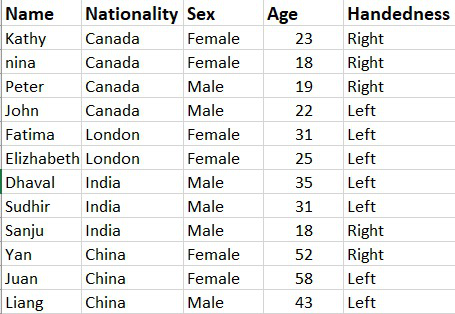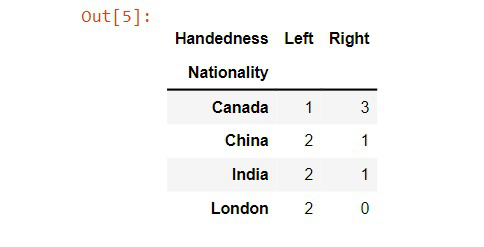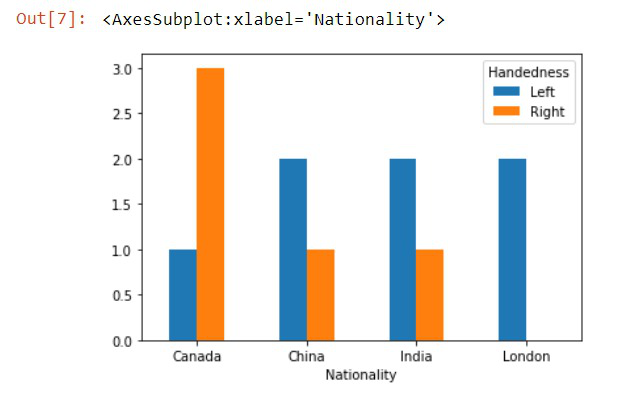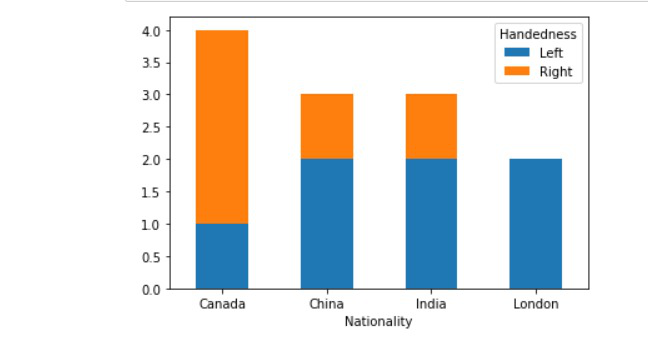# Using pandas crosstab to create a bar plot

• Difficulty Level : Basic
• Last Updated : 11 Jan, 2022

In this article, we will discuss how to create a bar plot by using pandas crosstab in Python. First Lets us know more about the crosstab, It is a simple cross-tabulation of two or more variables.

## What is cross-tabulation?

It is a simple cross-tabulation that help us to understand the relationship between two or more variable. It will give a clear understanding of the data and makes analysis easier.

Let us take an example if we take a data set of Handedness of people which includes peoples nationality, sex, age, and name. Suppose if we want to analyze the relationship between nationality and handedness of the peoples. Crosstab gives you the relationship between them.## Crosstab using pandas

Before creating the barplot we should create cross-tabulation using pandas.

Syntax: pandas.crosstab(index, columns, values=None, rownames=None, colnames=None, aggfunc=None, margins=False, margins_name=’All’, dropna=True, normalize=False

Code:

## Python

 `# importing the pandas library` `import` `pandas as pd`   `# Reading the csv file and storing it ` `# in a variable` `df ``=` `pd.read_csv(``'Data.csv'``)`   `# Crosstab function is called` `# 2 parameters are passed` `# The table is stored in a variable` `crosstb ``=` `pd.crosstab(df.Nationality, df.Handedness)`

Output:## Creating bar plots

Bar graphs are most used to compare between different groups or to track changes over time. Using bar plots to the crosstab is one of the efficient ways to conclude the crosstab and analyze them even better.

Syntax: DataFrame.plot.bar(x=None, y=None, **kwargs)

Code:

## Python3

 `# importing the pandas library` `import` `pandas as pd`   `# Reading the csv file and storing it in a variable` `df ``=` `pd.read_csv(``'Data.csv'``)`   `# Creating crosstab` `crosstb ``=` `pd.crosstab(df.Nationality, df.Handedness)`   `# Creating barplot` `barplot ``=` `crosstb.plot.bar(rot``=``0``)`

Output:## Stacked barplot

Here we will create a stacked barplot through dataframe by passing the stacked parameter as True.

Dataframe.plot(kind=”bar”, stacked = True, rot=0)

Code:

## Python

 `# importing the pandas library` `import` `pandas as pd`   `# Reading csv file` `df ``=` `pd.read_csv(``'Data.csv'``)`   `# Creating crosstab` `crosstb ``=` `pd.crosstab(df.Nationality, df.Handedness)`   `# Creating barplot` `pl ``=` `crosstb.plot(kind``=``"bar"``, stacked``=``True``, rot``=``0``)`

Output:## Creating bar plot using more than two variables from the crosstab

In the above example, we found the relationship between nationality and the handedness of the people. We can also create a crosstab with more than two values. We will implement this in the following example.

## Python3

 `# importing the module` `import` `pandas as pd`   `# reading the csv file` `df ``=` `pd.read_csv(``'Data.csv'``)`   `# Crosstab with three variables` `crosstb ``=` `pd.crosstab(df.Sex, [df.Nationality,` `                               ``df.Handedness])`   `# Bar ploting` `a ``=` `crosstb.plot(kind``=``'bar'``, rot``=``0``)` `a.legend(title``=``'Handedness'``, bbox_to_anchor``=``(``1``, ``1.02``), ` `         ``loc``=``'upper left'``)`

Output:My Personal Notes arrow_drop_up
Recommended Articles
Page :